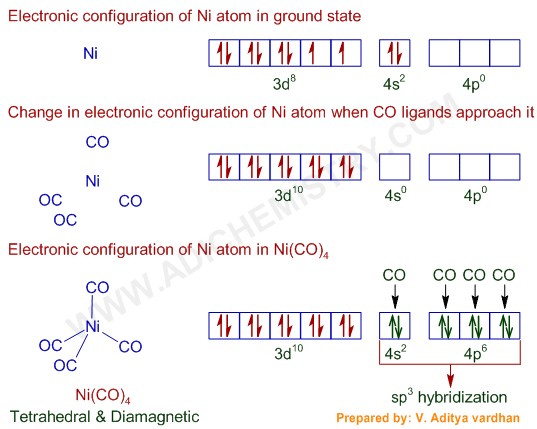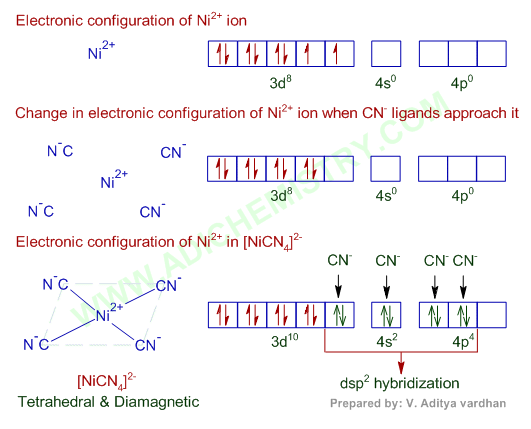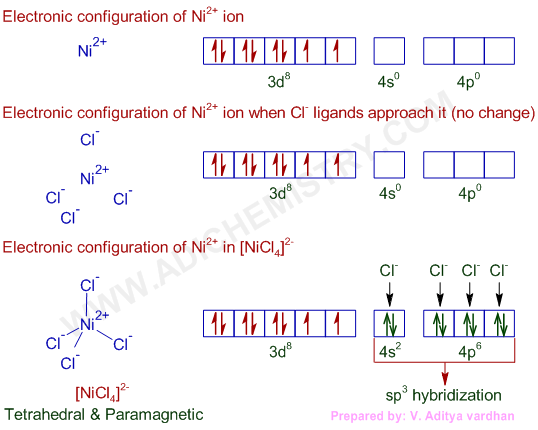# Ni(CO)4, [Ni(CN)4]2-, [Ni(Cl)4]2- HYBRIDIZATION-STRUCTURE- PARAMAGNETIC & DIAMAGNETIC EXAMPLES

## Question-1) Amongst Ni(CO)4, [Ni(CN)4]2- and NiCl42-:

(IIT JEE 1991)

a) Ni(CO)4 and NiCl42- are diamagnetic; and [Ni(CN)4] 2- is paramagnetic.

b) [Ni(CN)4]2- and NiCl42- are diamagnetic; and Ni(CO)4 is paramagnetic.

c) Ni(CO)4 and [Ni(CN)4]2- are diamagnetic; and NiCl42- is paramagnetic.

d) Ni(CO)4 is diamagnetic; [Ni(CN)4]2- and NiCl42- are paramagnetic.

Ni(CO)4 = Ni + 4CO

* The valence shell electronic configuration of ground state Ni atom is 3d8 4s2

* All of these 10 electrons are pushed into 3d orbitals and get paired up when strong field CO ligands approach Ni atom. The empty 4s and three 4p orbitals undergo sp3 hybridization and form bonds with CO ligands to give Ni(CO)4. Thus Ni(CO)4 is diamagnetic.KEY POINTS:

Hybridization of Ni(CO)4 : sp3

Shape & Structure (geometry) of Ni(CO)4: Tetrahedral

Magnetic nature: Diamagnetic (low spin)

[Ni(CN)4]2- = Ni2+ + 4CN-

* In [Ni(CN)4]2-, there is Ni2+ ion for which the electronic configuration in the valence shell is 3d8 4s0.

* In presence of strong field CN- ions, all the electrons are paired up. The empty 3d, 3s and two 4p orbitals undergo dsp2 hybridization to make bonds with CN- ligands in square planar geometry. Thus [Ni(CN)4]2- is diamagnetic. It is said to be a low spin inner orbital complex.KEY POINTS:

Hybridization of [Ni(CN)4]2- : dsp2

Shape of [Ni(CN)4]2-: Square planar

Magnetic nature: Diamagnetic (low spin)

NiCl42- = Ni2+ + 4Cl-

* Again in NiCl42-, there is Ni2+ ion, However, in presence of weak field Cl- ligands, NO pairing of d-electrons occurs. Therefore, Ni2+ undergoes sp3 hybridization to make bonds with Cl- ligands in tetrahedral geometry. As there are unpaired electrons in the d-orbitals, NiCl42- is paramagnetic and is referred to as a high spin outer orbital complex.KEY POINTS:

Hybridization  of Ni in [NiCl4]2- :sp3

Shape & Structure of [NiCl4]2- : Tetrahedral

Magnetic nature: Paramagnetic (low spin)

Conclusion:

The correct option is 'c'.

## Related questions

### Question-2) Among the following the lowest degree of paramagnetism per mole of the compound at 298 K will be shown by:

(IIT JEE 1988)

a) MnSO4.4H2O

b) CuSO4.5H2O

c) FeSO4.6H2O

d) NiSO4.6H2O

Logic & solution:

* In presence of water, which is a weak field ligand, the configurations of metal ions in hydrated compounds reflect those in isolated gaseous ions i.e., no pairing of electrons is possible as the interaction with water molecules is weak.

* All the metal ions in the above compounds are divalent and their outer shell electronic configurations are shown below.* The paramagnetic nature of a compound is proportional to the number of unpaired electrons in it. Mn2+ ion has more number of unpaired electrons. Hence MnSO4.4H2O shows greater paramagnetic nature.

Whereas there is only one unpaired electron in Cu2+ and hence CuSO4.5H2O shows lowest degree of paramagnetism.

Conclusion:

Correct option is: 'b'.

### Question-3) Among the following ions, which one will have highest paramagnetic behavior.

(IIT JEE 1993)

a) [Cr(H2O)6]3+

b) [Fe(H2O)6]2+

c) [Cu(H2O)6]2+

d) [Zn(H2O)6]2+

Logic & solution:

* As mentioned in previous question, the electronic configuration of metal ions is not much affected by weak field ligand water. Hence the electronic configurations of metal ions coordinated to water are same as in isolated ions.

* The outer shell electronic configurations of metal ions in the above complexes are shown below.* Fe2+ ion has more number of unpaired electrons. Hence [Fe(H2O)6]2+ is more paramagnetic.

Note: The charge on metal ions is equal to the charge on the complex since water is a neutral ligand.

Conclusion:

Correct option is: 'b'.

### Question-4) Transition metals show paramagnetic behavior. This is because of their :

(AFMC 2009)

A) high lattice energy

B) variable oxidation state

C) characteristic configuration

D) unpaired electrons

Correct option is: D

### Questions & Answers - Magnetic Nature of Ni complexes - MCQ

1) How do you calculate the magnetic moment of ions of transition elements?

2) What are the strong field and weak field ligands?

3) Why Ti(SO4)2 is diamagnetic?

4) What is the hybridization & structure of [CoCl4]2-? What is the magnetic nature of this compound?

5) Is CO paramagnetic or diamagnetic.....?

6) The geometry and magnetic behaviour of the complex Ni(CO)4 are , respectively.......

7) NiCl42- ion is paramagnetic. why?

8) The magnetic moment (spin only) of  NiCl42- is........?

9) The theory that can completely explain the nature of bonding in Ni(CO)4 is .........?

Question-10) The complex ion (NiCl4)2– is tetrahedral. How many unpaired electrons are there in the complex?

11) What is the magnetic moment of nickel ion in tetraammine nickel(ii) chloride, [Ni(NH3)4]Cl2?

12) Write the hybridisation and magnetic behaviour of the complex Ni(CO)4 .

13) Question - Amongst following, the lowest degree of paramagnetism per mole of the compound at 298 K will be shown by:

(a) MnSO4.4H2O

(b) CuSO4.5H2O

(c) FeSO4.6H2O

(d) NiSO4.6H2O

14) Which complex of nickel is paramagnetic in nature- (a) K2[NiCl4] (b) K2[Ni(CN)4] (c) K2[Ni(CO)4] (d) [Ni(NH3)4]Cl2 .

Question-15) Determine the number of unpaired electrons for ​[NiCl4]2-, known to be paramagnetic.

Answer: There are two unpaired electrons in [NiCl4]2-, a tetrahedral complex with sp3 hybridization in Ni.

16) Amongst the following, no. of species with tetrahedral geometry is : [Pd(CN)4]2–, [Ni(CN)4]2–, [PdCl4]2– and [NiCl4]2– .

Answer: Only one i.e. [NiCl4]2–

17) In [Ni(CO)4] and [NiCl4]2- species, the hybridization states of Ni atom/ion are respectively......

18) State whether the following statement is true or false: The molecular shape of Ni(CO)4 is not the same as that of [Ni(CN)4]2–.